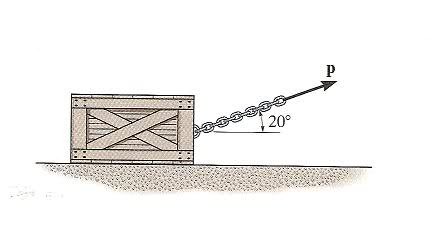# Kinetics, force and acceleration

red123

## Homework Statement

The crate has a mass of 80 kg and is being towed by a chain which is always directed at 20º from the horizontal has shown. Determine the crate's acceleration in t = 2 s if the coefficient of static friction is μs = 0.4, the coefficient of kinetic friction is μk = 0.3, and the towing force is P = (90t2) N, where t is in seconds.ΣF = ma

## The Attempt at a Solution

ΣFx = max
Pcos(20º) - μkmg = ma
84.57t2 - 235.44 = (80 kg)a

solving for a when t = 2
a = 1.29 m/s2

The answer at the back of my book is a = 1.75 m/s2. I think I'm supposed to consider that static friction force is greater than kinetic friction force, but I can't figure out how to do that.

Mentor
ΣFx = max
Pcos(20º) - μkmg = ma
The force of friction is μN, but N does not simply equal mg. Solve for N, taking into consideration that the applied force is at an angle.

red123
The force of friction is μN, but N does not simply equal mg. Solve for N, taking into consideration that the applied force is at an angle.

Then N = mg*cos(20°)?
But that gives a = 1.46 m/s2, not a = 1.75 m/s2.

Mentor
Then N = mg*cos(20°)?
How did you come to that conclusion?

To solve for N, add up the vertical components of all forces acting on the body. (N is one of those forces.) The sum must be zero, since the body is not accelerating vertically.

red123
Thank you, Doc Al.